## pandas常用操作_桥豆麻袋XQXQXQ的博客-程序员资料

python 数据分析学习笔记(二)

# 基于pandas的数据清洗和数据操作

### 1.处理丢失数据

##### 丢失数据类型：
• none
• np.nan(NaN)
``````type(None) ##对象类型不可以参与运算
type(np.nan)  #浮点型数据可以参与计算
``````

##### 处理空值的方法
• isnull+any
• notnull+all
``````data=DataFrame(data=np.random.randint(1,100,size=(7,5)))
data.iloc[2,3]=None
data.iloc[3,4]=np.nan
data.iloc[5,2]=np.nan
data.iloc[6,1]=None
````````````#isnull 结合any
#利用布尔索引，找到有空值的所有行
data.loc[data.isnull().any(axis=1)]
drop_index=data.loc[data.isnull().any(axis=1)].index
drop_index
#返回需要删除的行索引
Int64Index([2, 3, 5, 6], dtype='int64')
#删掉有空值的行
data.drop(labels=drop_index,axis=0)
#notnull 结合all
data=data.loc[data.notnull().all(axis=1)]
``````
• dropna
``````data.dropna(axis=0)
``````
• fullna
``````data.fillna(value=1)  #使用指定值填充空值 使用意义不大
#对空值进行赋值,使用ffill(从前一行或列)或者bfill(从后一行或列),axis确定行列
data.fillna(method='ffill',axis=1)
``````

### 2.级联

• pd.concat
• pd.append
``````import pandas as pd
import numpy as np
from pandas import DataFrame,Series

df1=DataFrame(data=np.random.randint(1,100,size=(5,3)),columns=['A','B','C'])
df2=DataFrame(data=np.random.randint(1,100,size=(5,3)),columns=['A','D','C'])
df=pd.concat((df1,df2),axis=0) #将df1与df2通过列进行连接
df=df1.append(df2)  # # 只能是列连接
``````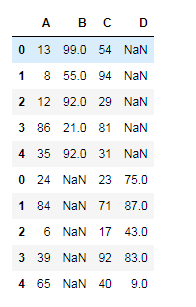### 3.合并

• concat
• merge (需要某一共同列进行合并) (重点)
``````df3=DataFrame({
'成绩':['A','B','C'],'name':['张三','李四','王五']})
df4=DataFrame({
'科目':['F','B','D'],'name':['张三','李四','王五']})
#根据姓名列进行两个表格的合并
#on不写的话,就使用两个表格中共有的列名进行合并(可以存在多个)
df=pd.merge(df3,df4,on='name')  #默认为内连接
df=pd.merge(df3,df4,on='name',how='left')  #左连接
#how的参数有outer(取并集),inner(取交集),left,right
``````

** replace**
1.单值替换:

2.多值替换:

### 4.映射操作

``````dic={
'name':['张三','李四','王五'],
'salary':[10000,20000,30000]}
data=DataFrame(data=dic)
``````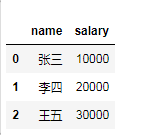``````dic={
'张三':'tom',
'李四':'join',
'王五':'yangm'}
data['name2']=data['name'].map(dic)
``````

map是Series的函数,只能由Series 调用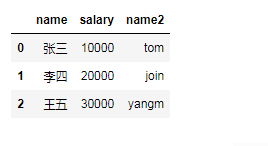### 4.运算操作

• apply (apply 用作dataframe中的计算工具)
将某种运算作用到df的每一行或者列中
``````###给df中的每列元素加上100
df=DataFrame(data=np.random.randint(1,100,size=(5,3)))
return values+100
``````
• applymap (将某种运算作用到df的每一个元素中)
``````df.applymap(add_)#没有axis的概念
``````

### 4.实现随机抽样

``````df=DataFrame(data=np.random.randint(1,100,size=(5,3)),columns=('A','B','C'))
#取确定的1,2,4行数据
df.take(indices=[1,2,4],axis=0)
#取前三行数据随机排序,再对前三列数据随机打乱
df.take(indices=np.random.permutation(3),axis=0).take(indices=np.random.permutation(3),axis=1)
``````

### 5.数据的分组操作

• groupby
• groups
``````dic={
'部门':['IT','人力','财务','IT','人力'],
'姓名':['张三','李四','王五','陈六','丁一'],
'salary':[30000,56000,7900,8000,9000]}
df=DataFrame(dic)
``````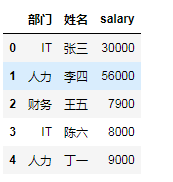``````#根据部门分组
df.groupby(by='部门').groups
#分组聚合,sum(),mean()
df.groupby(by='部门').mean()  #会聚合所有能聚合的列,如果计算单一列,可做col限制
#agg用于将分组以后的结果做多种不同方式的聚合
df.groupby(by='部门')['salary'].agg(['min','max'])
#将计算的均值以新一列加入到原表中,可以使用映射
df['均值']=df['部门'].map(df.groupby(by='部门')['salary'].mean())

``````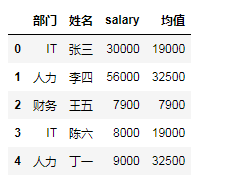``````def salary_new(s):
return s*1.1
df['new_s']=df.groupby(by='姓名')['salary'].apply(salary_new)
``````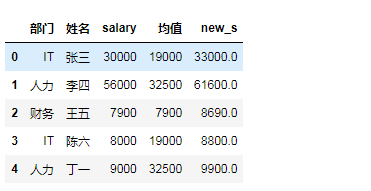### 6.读取数据库里的数据

``````import pymysql
conn=pymysql.connect(host='172......2',user='c.....o',passwd='l......c',db='t...t',charset='utf8')
``````

### 7.pandas实现透视表和交叉表

``````#对老师和群状态做'群总发言量','群成员人数','群活跃人数'的数据汇总,计算求和
sql.pivot_table(index=['老师','群状态'],values=['群总发言量','群成员人数','群活跃人数'],aggfunc=sum)
#交叉表,计算每个老师在每个群状态下的群活跃人数的和,没有的补0
sql.pivot_table(index=['群状态'],values=['群活跃人数'],columns=['老师'],aggfunc=sum,fill_value=0)
``````

pd.crosstab(index=sql[‘群状态’],columns=sql[‘老师’])

### 8.pandas实现DataFrame 分列

``````#将col2列根据"-"分列,取第一列
df['col1']=df['col2'].map(lambda x:x.split("-"))
``````

### ubuntu16.04安装anaconda（python2.7 版本）_深夜虫鸣的博客-程序员资料

1.下载anaconda安装文件，官网速度较慢，建议清华开源软件镜像站下载： https://mirrors.tuna.tsinghua.edu.cn/help/anaconda/ 2.用终端进入anaconda安装文件所在文件夹路径（cd xxx） 3. 在终端输入bash xxx(xxx为anaconda安装文件名 4. 不断按enter键是命令窗口显示的内容来到协议末尾，输入yes，然后

### ios 中如何应对UIScrollView快速滑动（暴力用户，暴力测试）_SayKingLing的博客-程序员资料

1.实现UIScrollViewDelegate开始滑动：- (void)scrollViewWillBeginDecelerating:(UIScrollView *)scrollView滑动过程：- (void)scrollViewDidScroll:(UIScrollView *)scrollView滑动结束：- (void)scrollViewDidEn

### git 如何同步本地、远程的分支和tag信息_I am Big Boss~~的博客-程序员资料_git 同步远程tag

git 如何在本地同步远程分支和tag1.git如何同步本地分支与远程origin的分支问题场景：同事A创建了本地分支branchA并push到了远程-&amp;amp;amp;gt;同事B在本地拉取(git fetch)了和远程branchA同步的本地分支branchA-&amp;amp;amp;gt;同事A开发完成将远程分支branchA删除（远程仓库已经不存在分支branchA）-&amp;amp;amp;gt;同事B用git fetch同步远端分支，gi...

### YApi Mock功能远程代码执行漏洞复现_yyyyzzzllll的博客-程序员资料

YApi Mock功能远程代码执行漏洞复现漏洞描述API接口管理平台是国内某旅行网站的大前端技术中心开源项目，使用mock数据/脚本作为中间交互层，为前端后台开发与测试人员提供更优雅的接口管理服务，该系统被国内较多知名互联网企业所采用。 YApi 是高效、易用、功能强大的 api 管理平台。但因为大量用户使用 YAPI的默认配置并允许从外部网络访问 YApi服务，导致攻击者注册用户后，即可通过 Mock功能远程执行任意代码。影响版本YApi7月7日前版本，即版本&lt;=1.92（后文有说明）环境

### leedcode题解-贪心_h_wzy的博客-程序员资料

1、分配饼干455. Assign Cookies (Easy)题目描述：每个孩子都有一个满足度，每个饼干都有一个大小，只有饼干的大小大于等于一个孩子的满足度，该孩子才会获得满足。求解最多可以获得满足的孩子数量。Input: [1,2], [1,2,3] Output: 2思路：对饼干大小、孩子的满足度排序，尽最大可能满足更多的孩子；class Solution {publ...